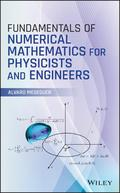John Wiley & Sons Fundamentals of Numerical Mathematics for Physicists and EngineersIntroduces the fundamentals of numerical mathematics and illustrates its applications to a wide vari.. Product #: 978-1-119-42567-0 Regular price: \$113.33 \$113.33 Auf Lager

# Fundamentals of Numerical Mathematics for Physicists and Engineers1. Auflage Juli 2020
400 Seiten, Hardcover
Wiley & Sons Ltd

ISBN: 978-1-119-42567-0
John Wiley & Sons

Jetzt kaufen

### Preis: 119,00 €

Preis inkl. MwSt, zzgl. Versand

Introduces the fundamentals of numerical mathematics and illustrates its applications to a wide variety of disciplines in physics and engineering

Applying numerical mathematics to solve scientific problems, this book helps readers understand the mathematical and algorithmic elements that lie beneath numerical and computational methodologies in order to determine the suitability of certain techniques for solving a given problem. It also contains examples related to problems arising in classical mechanics, thermodynamics, electricity, and quantum physics.

Fundamentals of Numerical Mathematics for Physicists and Engineers is presented in two parts. Part I addresses the root finding of univariate transcendental equations, polynomial interpolation, numerical differentiation, and numerical integration. Part II examines slightly more advanced topics such as introductory numerical linear algebra, parameter dependent systems of nonlinear equations, numerical Fourier analysis, and ordinary differential equations (initial value problems and univariate boundary value problems). Chapters cover: Newton's method, Lebesgue constants, conditioning, barycentric interpolatory formula, Clenshaw-Curtis quadrature, GMRES matrix-free Krylov linear solvers, homotopy (numerical continuation), differentiation matrices for boundary value problems, Runge-Kutta and linear multistep formulas for initial value problems. Each section concludes with Matlab hands-on computer practicals and problem and exercise sets. This book:
* Provides a modern perspective of numerical mathematics by introducing top-notch techniques currently used by numerical analysts
* Contains two parts, each of which has been designed as a one-semester course
* Includes computational practicals in Matlab (with solutions) at the end of each section for the instructor to monitor the student's progress through potential exams or short projects
* Contains problem and exercise sets (also with solutions) at the end of each section

Fundamentals of Numerical Mathematics for Physicists and Engineers is an excellent book for advanced undergraduate or graduate students in physics, mathematics, or engineering. It will also benefit students in other scientific fields in which numerical methods may be required such as chemistry or biology.

Preface xi

Acknowledgments xv

Part I 1

1 Solution Methods for Scalar Nonlinear Equations 3

1.1 Nonlinear Equations in Physics 3

1.2 Approximate Roots: Tolerance 5

1.2.1 The Bisection Method 6

1.3 Newton's Method 10

1.4 Order of a Root-Finding Method 13

1.5 Chord and Secant Methods 16

1.6 Conditioning 18

1.7 Local and Global Convergence 20

Problems and Exercises 24

2 Polynomial Interpolation 29

2.1 Function Approximation 29

2.2 Polynomial Interpolation 30

2.3 Lagrange's Interpolation 33

2.3.1 Equispaced Grids 37

2.4 Barycentric Interpolation 39

2.5 Convergence of the Interpolation Method 43

2.5.1 Runge's Counterexample 46

2.6 Conditioning of an Interpolation 49

2.7 Chebyshev's Interpolation 54

Problems and Exercises 60

3 Numerical Differentiation 63

3.1 Introduction 63

3.2 Differentiation Matrices 66

3.3 Local Equispaced Differentiation 72

3.4 Accuracy of Finite Differences 75

3.5 Chebyshev Differentiation 80

Problems and Exercises 84

4 Numerical Integration 87

4.1 Introduction 87

4.3 Accuracy of Quadrature Formulas 98

4.5 Integration of Periodic Functions 112

4.6 Improper Integrals 115

4.6.1 Improper Integrals of the First Kind 116

4.6.2 Improper Integrals of the Second Kind 119

Problems and Exercises 125

Part II 129

5 Numerical Linear Algebra 131

5.1 Introduction 131

5.2 Direct Linear Solvers 132

5.2.1 Diagonal and Triangular Systems 133

5.2.2 The Gaussian Elimination Method 135

5.3 LU Factorization of a Matrix 140

5.3.1 Solving Systems with LU 145

5.3.2 Accuracy of LU 147

5.4 LU with Partial Pivoting 150

5.5 The Least Squares Problem 160

5.5.1 QR Factorization 162

5.5.2 Linear Data Fitting 173

5.6 Matrix Norms and Conditioning 178

5.7 Gram-Schmidt Orthonormalization 183

5.7.1 Instability of CGS: Reorthogonalization 187

5.8 Matrix-Free Krylov Solvers 193

Problems and Exercises 204

6 Systems of Nonlinear Equations 209

6.1 Newton's Method for Nonlinear Systems 210

6.2 Nonlinear Systems with Parameters 220

6.3 Numerical Continuation (Homotopy) 224

Problems and Exercises 232

7 Numerical Fourier Analysis 235

7.1 The Discrete Fourier Transform 235

7.1.1 Time-Frequency Windows 243

7.1.2 Aliasing 246

7.2 Fourier Differentiation 251

Problems and Exercises 258

8 Ordinary Differential Equations 261

8.1 Boundary Value Problems 262

8.1.1 Bounded Domains 262

8.1.2 Periodic Domains 275

8.1.3 Unbounded Domains 277

8.2 The Initial Value Problem 279

8.2.1 Runge-Kutta One-Step Formulas 281

8.2.2 Linear Multistep Formulas 287

8.2.3 Convergence of Time-Steppers 297

8.2.4 A-Stability 301

8.2.5 A-Stability in Nonlinear Systems: Stiffness 315

Problems and Exercises 330

Solutions to Problems and Exercises 335

Glossary of Mathematical Symbols 367

Bibliography 369

Index 373
ALVARO MESEGUER, PHD, is Associate Professor at the Department of Physics at Polytechnic University of Catalonia (UPC BarcelonaTech), Barcelona, Spain, where he teaches Numerical Methods, Fluid Dynamics, and Mathematical Physics to advanced undergraduates in Engineering Physics and Mathematics. He has published more than 30 articles in peer-reviewed journals within the fields of computational fluid dynamics, and nonlinear physics.